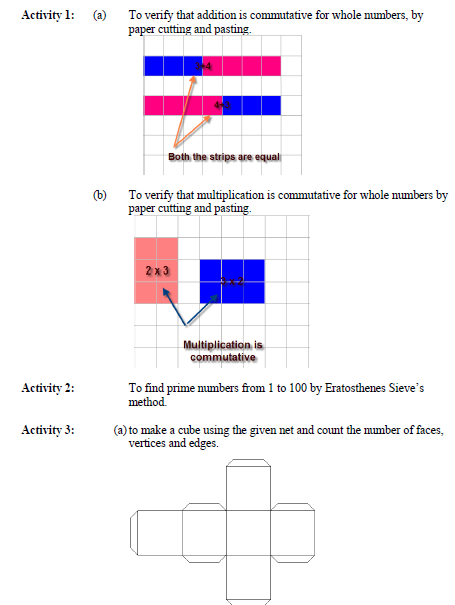# CBSE Class 6 Maths Activities

Read and download CBSE Class 6 Maths Activities chapter in NCERT book for Class 6 Mathematics. You can download latest NCERT eBooks for 2022 chapter wise in PDF format free from Studiestoday.com. This Mathematics textbook for Class 6 is designed by NCERT and is very useful for students. Please also refer to the NCERT solutions for Class 6 Mathematics to understand the answers of the exercise questions given at the end of this chapter

## Activities Class 6 Mathematics NCERT

Class 6 Mathematics students should refer to the following NCERT Book chapter Activities in standard 6. This NCERT Book for Grade 6 Mathematics will be very useful for exams and help you to score good marks

### Activities NCERT Class 6Please refer to the link below - CBSE Class 6 Maths Activities

 NCERT Class 6 Maths Knowing our Numbers
 NCERT Class 6 Maths Mensuration
 NCERT Class 6 Maths Algebra
 NCERT Class 6 Maths Ratio and Proportion
 NCERT Class 6 Maths Symmetry
 NCERT Class 6 Maths Practical Geometry
 NCERT Class 6 Maths Whole Numbers
 NCERT Class 6 Maths Playing with Numbers
 NCERT Class 6 Maths Basic Geometrical Ideas
 NCERT Class 6 Maths Understanding Elementary Shapes
 NCERT Class 6 Maths Integers
 NCERT Class 6 Maths Fractions
 NCERT Class 6 Maths Decimals
 NCERT Class 6 Maths Data Handling
 NCERT Class 6 Maths Answers to all Chapters
 NCERT Class 6 Maths Brain Teasers